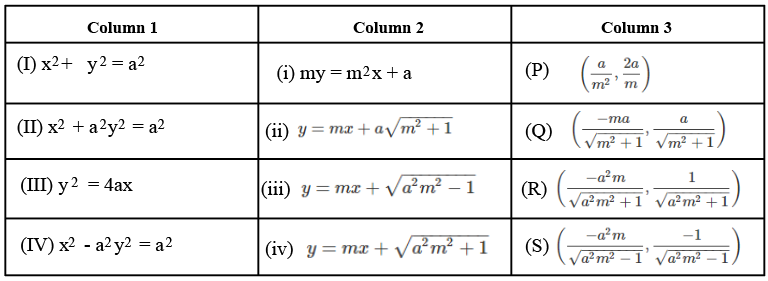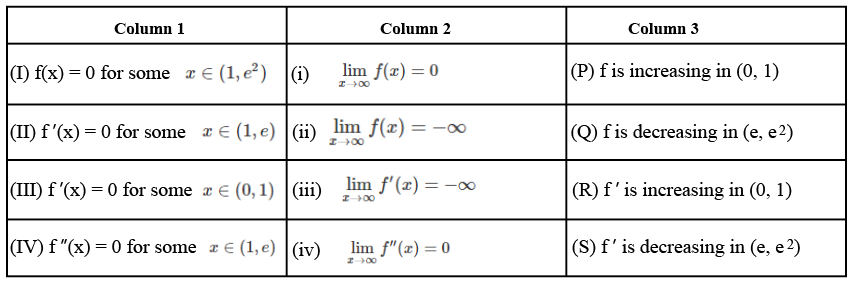Instructions

Answer Questions by appropriately matching the information given in the three columns of the following table.

Columns 1, 2 and 3 contain conics, equations of tangents to the conics and points of contact, respectively.Question 51

# The tangent to a suitable conic (Column 1) at $$\left(\sqrt{3}, \frac{1}{2}\right)$$ is found to be $$\sqrt{3}x + 2y = 4$$, then which of the following options is the only CORRECT combination?

Instructions

Answer the Questions by appropriately matching the information given in the three columns of the following table.

Let $$f(x) = x + \log_e x - x \log_e x, x \in (0, \infty)$$.
Column 1 contains information about zeros of $$f(x), f'(x)$$ and $$f''(x)$$.
Column 2 contains information about the limiting behavior of $$f(x), f'(x)$$ and $$f''(x)$$ at infinity.
Column 3 contains information about increasing/decreasing nature of $$f(x)$$ and $$f'(x)$$.Question 52

Question 53

Question 54

OR